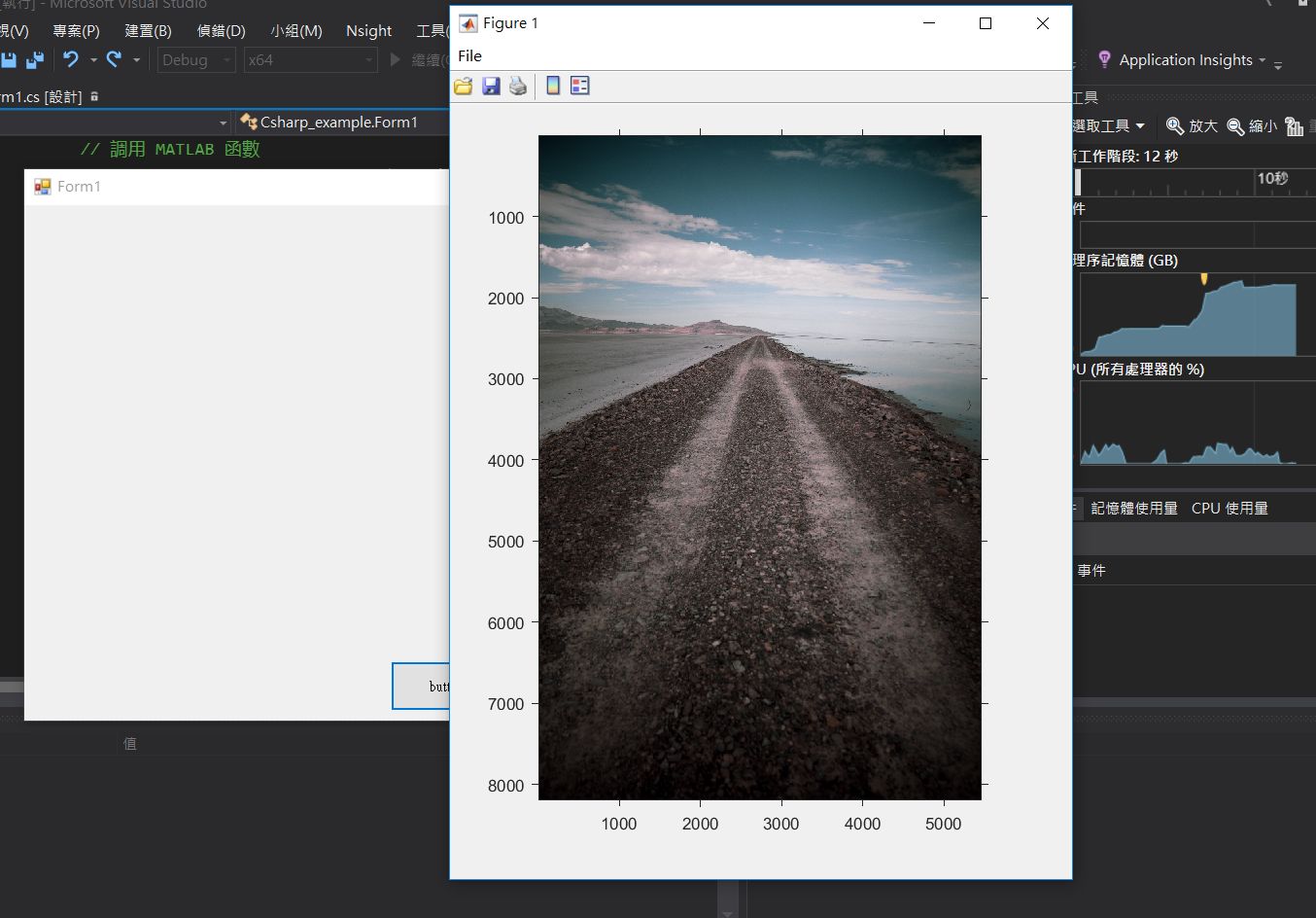### 【Mixing】在 C# 與 MATLAB 間交換影像資料

• 450
• 0
• 2019-06-05

MATLAB​ 軟體版本：
MATLAB                            Version 9.6      (R2019a)
MATLAB Compiler         　Version 7.0.1   (R2019a)
MATLAB Compiler SDK    Version 6.6.1   (R2019a)

C# 作業平台：
Visual Studio 2015 community
emgucv 4.0.1.3373C:\Emgu\emgucv-windesktop 4.0.1.3373\bin
C:\Emgu\emgucv-windesktop 4.0.1.3373\libs\x64

## 情境1：在 MATLAB 中使用 C# 讀取影像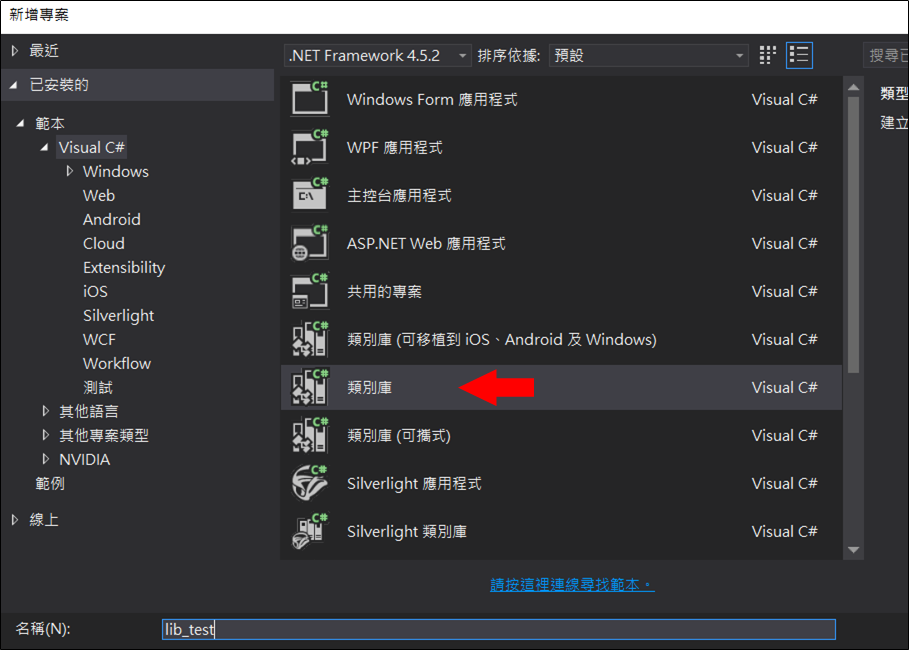// C# code
// 說明：供 MATLAB 使用的類別庫
//
// 2019.05.23 Shayne

using Emgu.CV;
using Emgu.CV.Structure;

// 請自行決定 namespace 的名稱
namespace lib_test
{
public class Class1
{
{
return new Image<Bgr, byte>(file_path);
}
}
}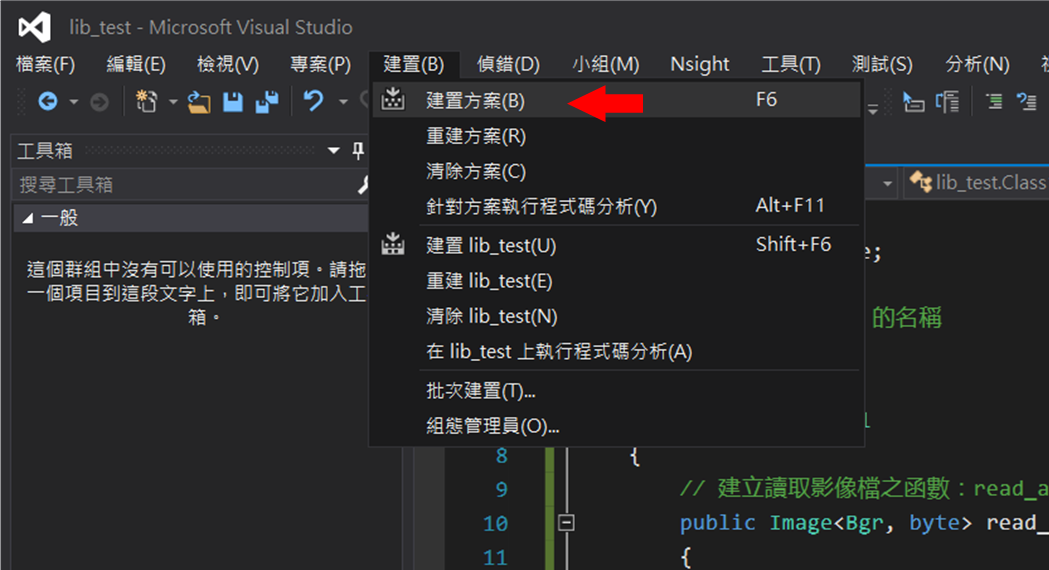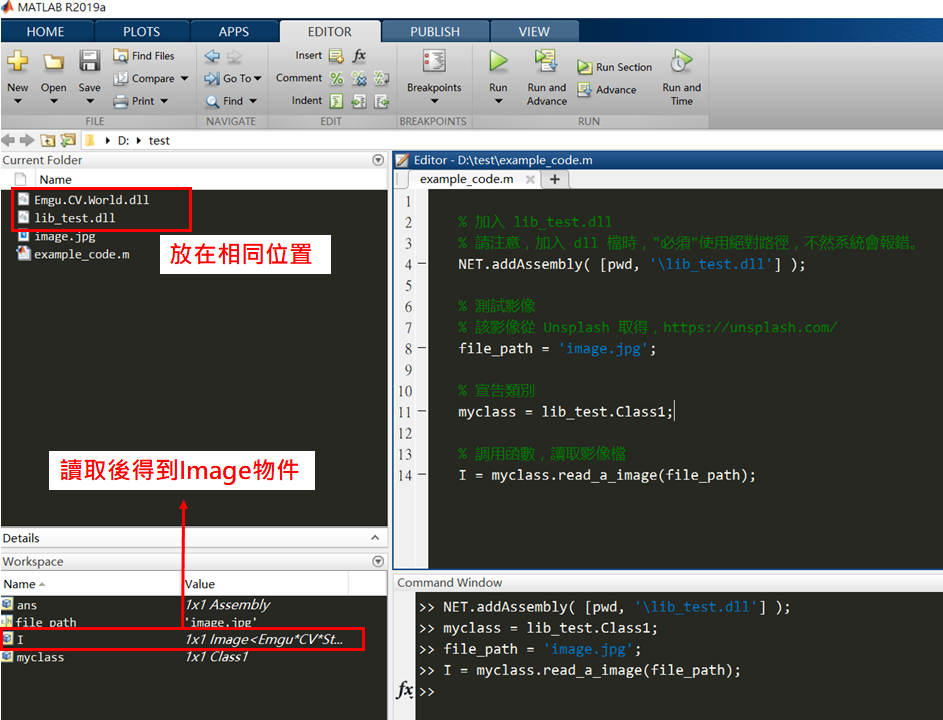% MATLAB code
%
% 2019.05.23 Shayne

% 加入 lib_test.dll
% 請注意，加入 dll 檔時，"必須"使用絕對路徑，不然系統會報錯。

% 測試影像
% 該影像從 Unsplash 取得，https://unsplash.com/
file_path = 'image.jpg';

% 宣告類別
myclass = lib_test.Class1;

% 調用函數，讀取影像檔
I = myclass.read_a_image(file_path);

%% 二次加工

% 取出I.Data，並轉成 uint8 格式
I1 = uint8(I.Data);

% 翻轉 BGR 為 RGB
I1 = flip(I1, 3);

imshow(I1)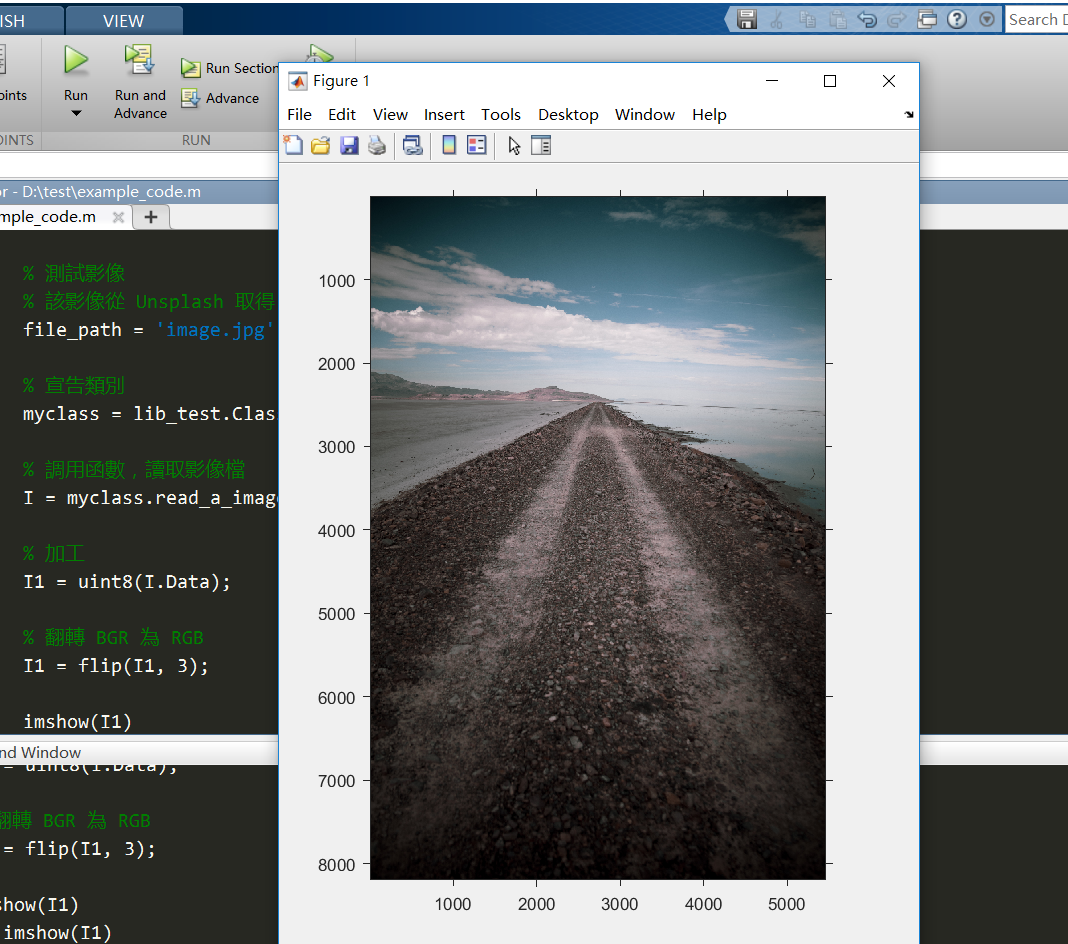## 情境2：在 C# 中使用 MATLAB 讀取影像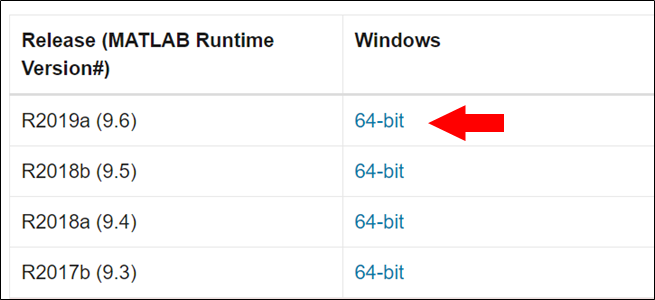C:\Program Files\MATLAB\MATLAB Runtime\v96\runtime\win64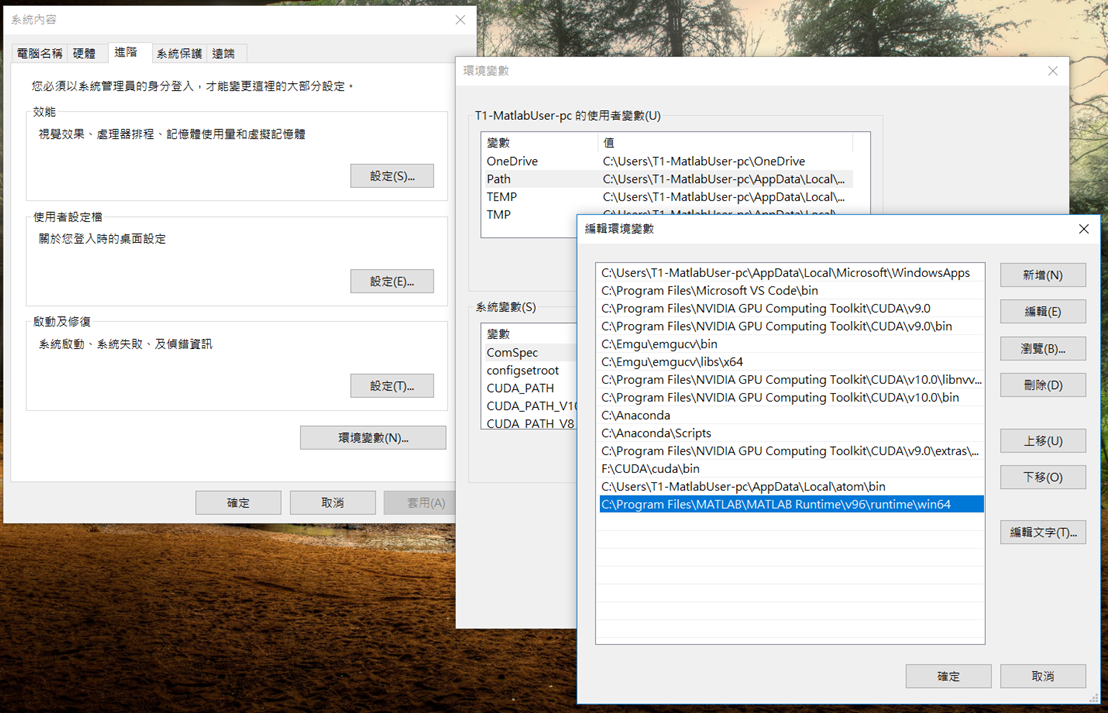function I = matlab_read_image(file_path)
% MATLAB code
% 說明：不簡單的讀檔程式
%
% 2019.05.23 Shayne

% 回傳給 C# 使用時，必須先調整影像格式：
% 1. 轉 RGB 為 BGR
I = flip(I, 3);
% 2. 調整陣列規格從 [R, C, 3] 為 [C, 3, R]
I = permute(I, [2, 3, 1]);

permute 功能為重新排列N 維數組的維度，語法為：B = permute(A,order)。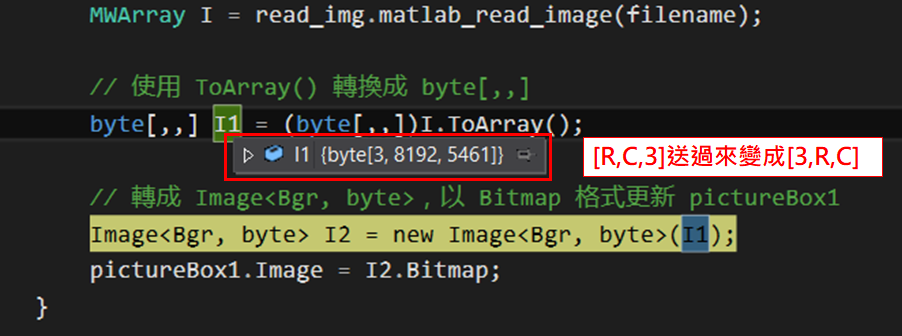註：原圖大小為：8192 x 5461 x 3 pixel。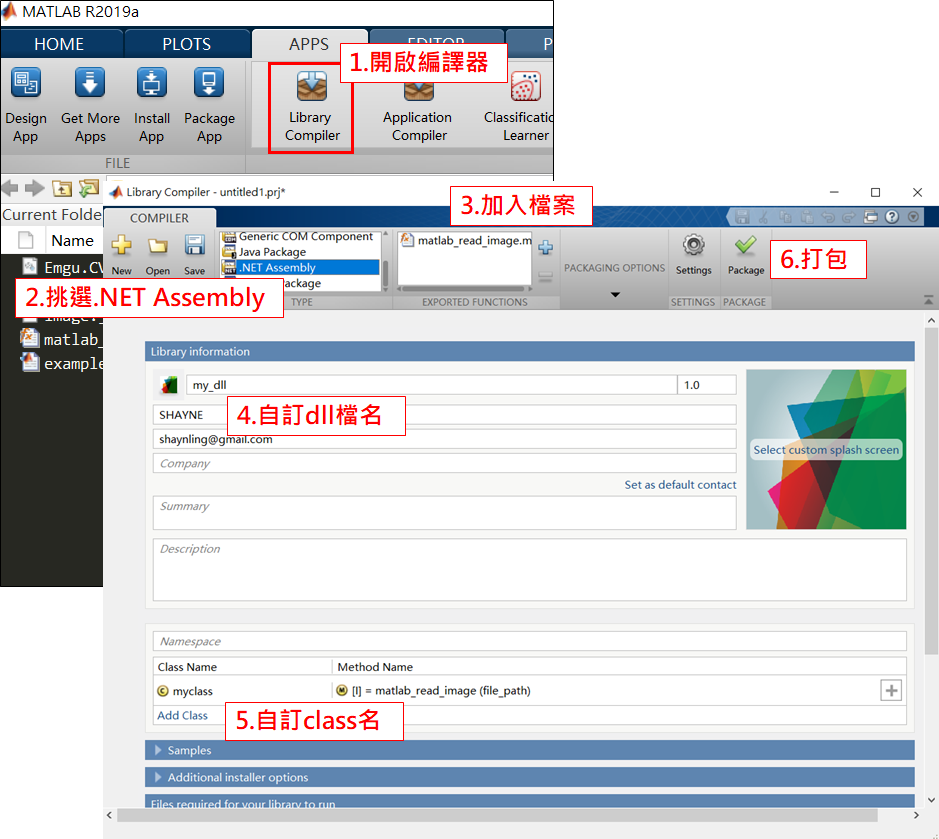.\my_dll\for_redistribution_files_only\my_dll.dll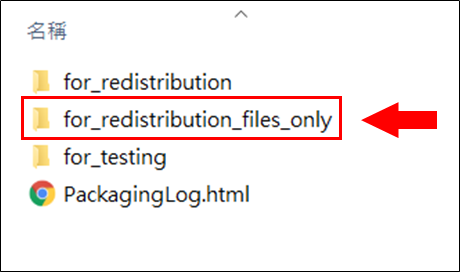================== 我是分隔線 ==================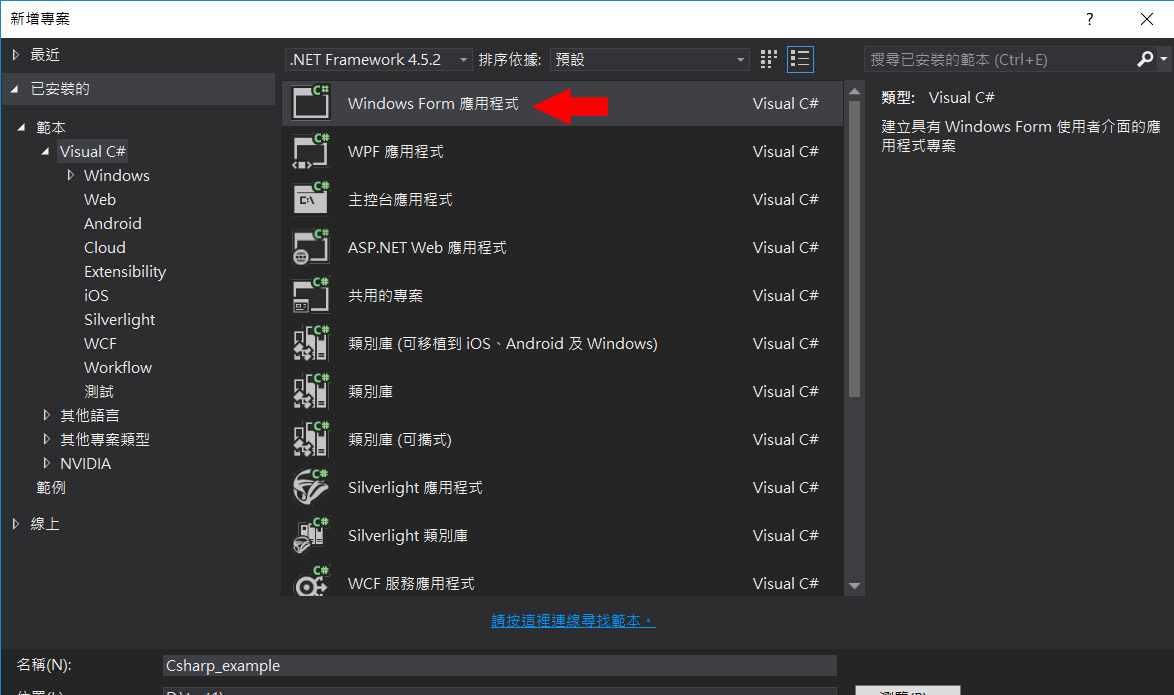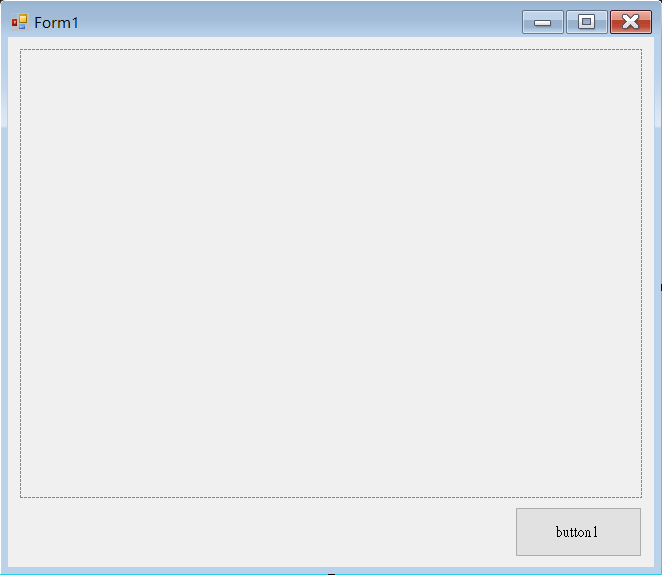1. Emgu.CV.World.dll
如同前一節所述，位於：
C:\Emgu\emgucv-windesktop 4.0.1.3373\bin\Emgu.CV.World.dll

2. MWArray.dll
這個是 MATLAB 提供的，位置在：
C:\Program Files\MATLAB\R2019a\toolbox\dotnetbuilder\bin\win64\v4.0\MWArray.dll

3. my_dll.dll
至於這個嘛...應該不用夏恩多說，就自己放哪兒自己明白。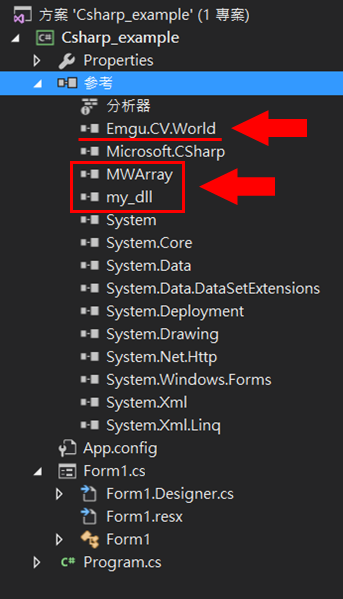MATLAB 在 2016 年之後發行的版本，已經不支援 x86 的組態，請特別小心。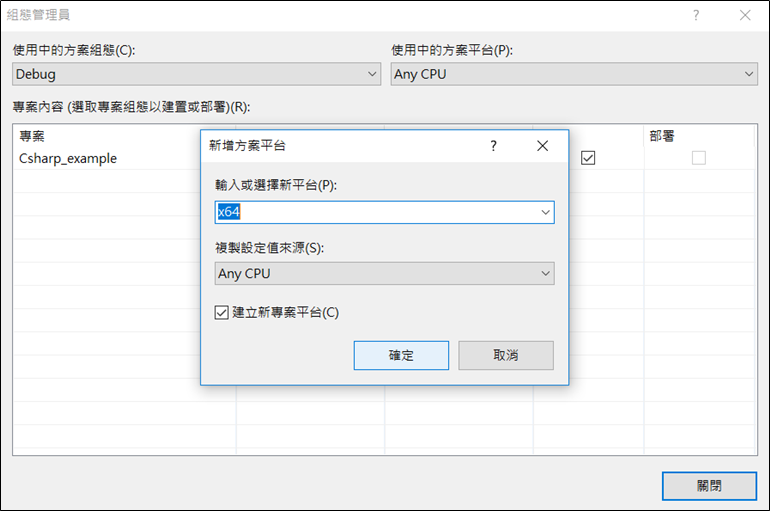(檔名)   > (類別名)  > (函數名)

1. using 檔名
2. 類別名(myclass)宣告

// C# code
// 說明：調用 MATLAB 函數說明
//
// 2019.05.23 Shayne

using System;
using System.Windows.Forms;

// Emgu 相關檔案
using Emgu.CV;
using Emgu.CV.Structure;

// MATLAB 相關檔案
using MathWorks.MATLAB.NET.Arrays;

// 自製函式庫
using my_dll;

namespace Csharp_example
{
public partial class Form1 : Form
{
public Form1()
{
InitializeComponent();
}

// 宣告自定義類別
myclass my_cls = new myclass();

private void button1_Click(object sender, EventArgs e)
{
// 開檔
string filename = OpenFile();

// 調用 MATLAB 函數

// 使用 ToArray() 轉換成 byte[,,]
byte[,,] I1 = (byte[,,])I.ToArray();

// 轉成 Image<Bgr, byte>，以 Bitmap 格式更新 pictureBox1
Image<Bgr, byte> I2 = new Image<Bgr, byte>(I1);
pictureBox1.Image = I2.Bitmap;
}

private string OpenFile()
{
OpenFileDialog dialog = new OpenFileDialog();
dialog.Title = "Select file";
dialog.InitialDirectory = @".\";
dialog.Filter = "影像檔|*.jpg";

string filename;
if (dialog.ShowDialog() == DialogResult.OK && dialog.FileName != null)
filename = dialog.FileName;
else
filename = null;

return filename;
}
}
}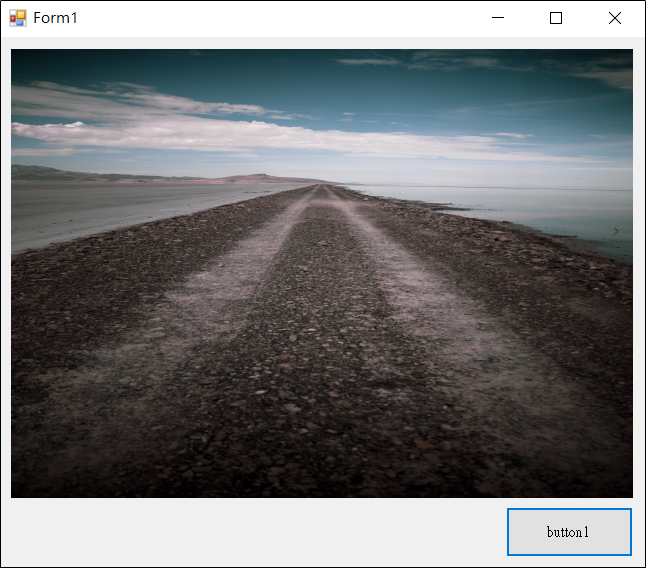## 再延伸

function matlab_show_image(I)
% MATLAB code
% 說明：不簡單的秀圖程式
%
% 2019.05.23 Shayne

% 承接 C# 影像時，必須先調整影像格式：

% 1. 調整陣列大小從 [C, 3, R] 為 [R, C, 3]
I = permute(I, [3, 1, 2]);

% 1. 轉 BGR 為 RGB
I = flip(I, 3);

imshow(I)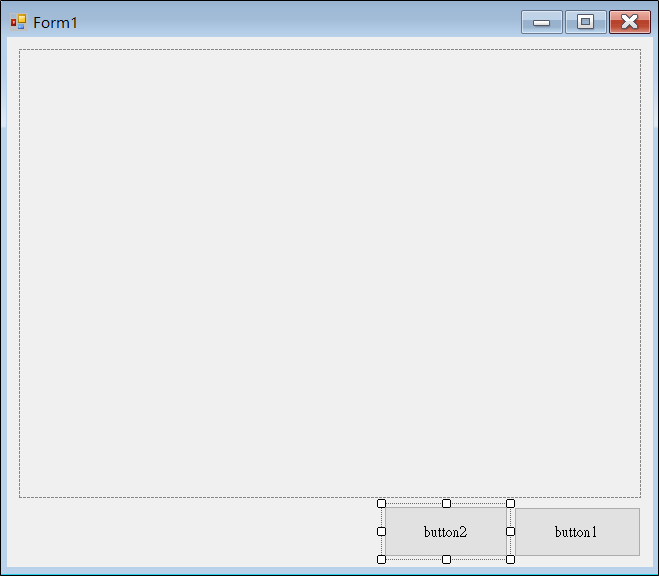private void button2_Click(object sender, EventArgs e)
{
// 開檔
string filename = OpenFile();

// 讀檔
Image<Bgr, byte> I = new Image<Bgr, byte>(filename);

// 調用 MATLAB 函數
my_cls.matlab_show_image((MWNumericArray)I.Data);
}

1. 使用 Image<Bgr, byte> 讀檔，而非 Bitmap。
理由就是 Bitmap 沒有 Data 屬性，又慢又難用！

2. 用 (MWNumericArray) 語法將 Data 屬性做轉換，即可傳送。
如果沒有轉型這個步驟，程式是不會動的。